Healthy Ride Network Analysis

This post explores the Healthy Ride dataset using network analysis in R.

This is my second post about the Healthy Ride bike service in Pittsburgh. You can find the first post here, where I did some exploratory analysis of the data.

First, load the R packages we will be using:

library(tidyverse)
library(ggraph)
library(igraph)
library(lubridate)
library(viridis)
library(stringr)
library(knitr)
library(kableExtra)

Then set the theme to format the plots:

theme_set(theme_graph())

Set the seed for the random number generator so the charts look the same every time they are run:

set.seed(12345)

Load the data from the WPRDC (hosted on my GitHub page):

data <- read_csv("https://raw.githubusercontent.com/conorotompkins/healthy_ride/master/data/data.csv")
## Parsed with column specification:
## cols(
##   Trip id = col_integer(),
##   Starttime = col_character(),
##   Stoptime = col_character(),
##   Bikeid = col_integer(),
##   Tripduration = col_integer(),
##   From station id = col_integer(),
##   From station name = col_character(),
##   To station id = col_integer(),
##   To station name = col_character(),
##   Usertype = col_character()
## )

Again, we need to format the data and the column names to make them more useful for analysis. Since this is a repeat of the script from the last post, I will just do it all in one go:

colnames(data) <- tolower(colnames(data))
colnames(data) <- gsub(" ", "_", colnames(data))

data_long <- data %>%
rename(start_date_time = starttime,
stop_date_time = stoptime) %>%
gather(date_time_type, date_time, c(start_date_time, stop_date_time)) %>%
select(date_time_type, date_time, everything()) %>%
mutate(date_time_2 = date_time) %>%
separate(date_time, " ", into = c("date", "time")) %>%
mutate(id = row_number(),
date = mdy(date),
year = year(date),
month = month(date, label = TRUE),
week = week(date),
time = hm(time),
hour = hour(time),
wday = wday(date, label = TRUE),
is_weekday = ifelse(wday %in% c("Mon", "Tues", "Wed", "Thurs", "Fri"), "weekday", "weekend"),
yday = yday(date),
mday = mday(date)) %>%
mutate(trip_duration = (tripduration / 60) / 60) %>%
gather(station_id_type, station_id, c(from_station_id, to_station_id)) %>%
gather(station_name_type, station_name, c(from_station_name, to_station_name)) %>%
select(date_time_type,
is_weekday,
date,
year,
month,
time,
hour,
wday,
yday,
mday,
date_time_2,
station_id_type,
station_id,
station_name_type,
station_name,
everything())

The data is currently arranged to be “long”, which means that the data that shows where a trip started and ended are in the same column (station_name_type)

unique(data_long$station_name_type) ##  "from_station_name" "to_station_name" data_long %>% select(station_name_type, station_name, date_time_2) %>% head() %>% kable("html") %>% kable_styling() station_name_type station_name date_time_2 from_station_name 37th St & Butler St 5/31/2015 6:54 from_station_name 37th St & Butler St 5/31/2015 6:57 from_station_name 42nd St & Butler St 5/31/2015 6:58 from_station_name Forbes Ave & Market Square 5/31/2015 7:08 from_station_name 37th St & Butler St 5/31/2015 7:15 from_station_name Forbes Ave & Market Square 5/31/2015 7:16 This makes analyzing where a trip started and ended difficult. To make the data more useful for network analysis, we need to make it a bit wider. This code splits the contents of the station_name_type column into two separate columns: from_station_name and to_station_name. data_wide <- data_long %>% spread(station_name_type, station_name) %>% select(from_station_name, to_station_name, everything()) data_wide[1:10, 1:3] %>% kable("html") %>% kable_styling() from_station_name to_station_name date_time_type 37th St & Butler St Forbes Ave & Market Square start_date_time 37th St & Butler St 37th St & Butler St start_date_time 42nd St & Butler St 42nd St & Butler St start_date_time Forbes Ave & Market Square Forbes Ave & Market Square start_date_time 37th St & Butler St 37th St & Butler St start_date_time Forbes Ave & Market Square Forbes Ave & Market Square start_date_time 10th St & Penn Ave (David L. Lawrence Convention Center) 10th St & Penn Ave (David L. Lawrence Convention Center) start_date_time 42nd St & Butler St Forbes Ave & Market Square start_date_time 42nd St & Butler St Forbes Ave & Market Square start_date_time Forbes Ave & Grant St Liberty Ave & Stanwix St start_date_time To start, lets look at the entire network. The loops indicate that the trip began and ended at the same station. simple_network <- data_wide %>% count(from_station_name, to_station_name, sort = TRUE) simple_network <- simple_network %>% graph_from_data_frame(directed = TRUE) ggraph(simple_network) + geom_edge_fan(aes(edge_alpha = n)) + geom_edge_loop(aes(edge_alpha = n)) + geom_node_point() + scale_edge_alpha_continuous("Number of rides", range = c(.1, 1))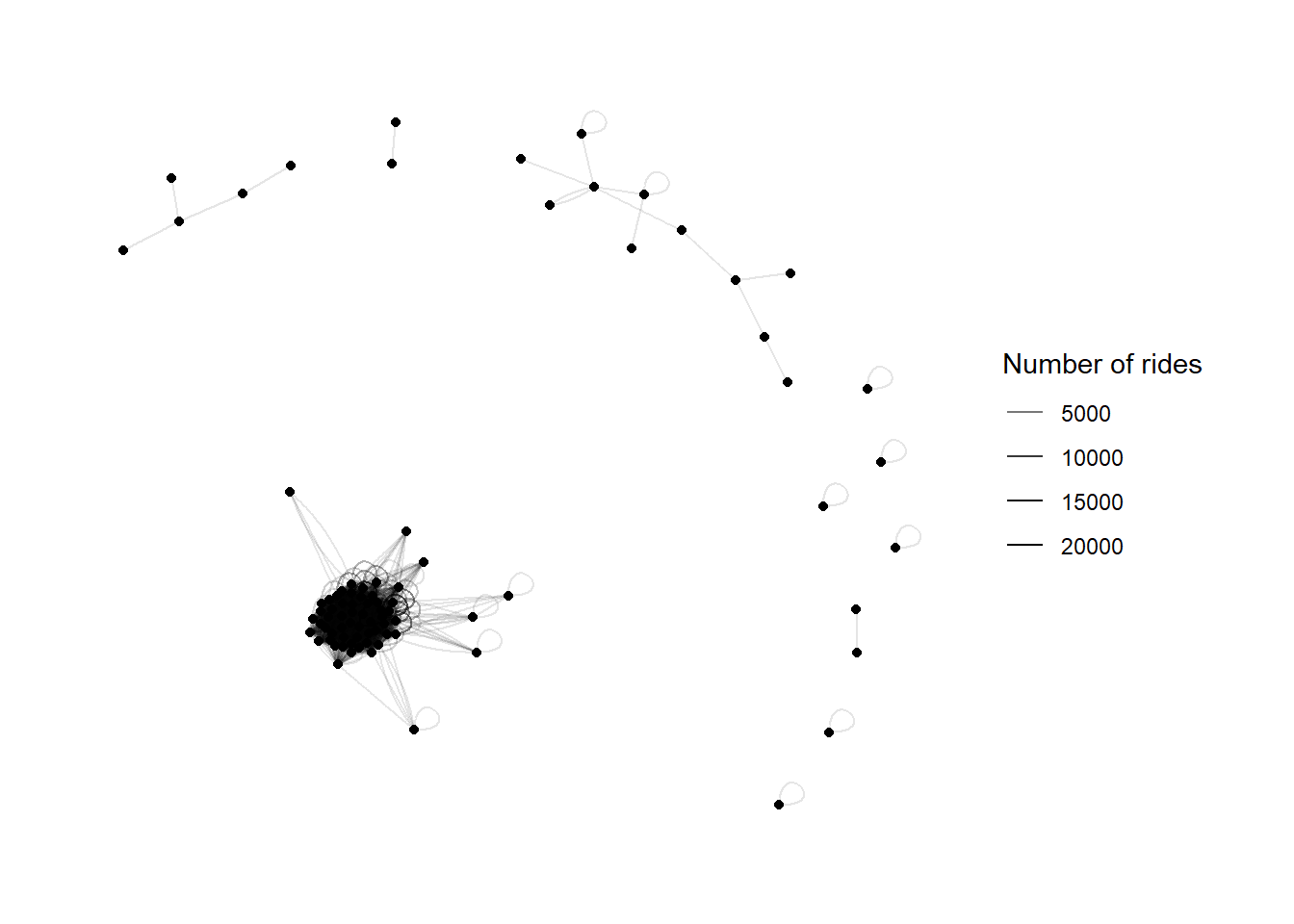This is a bit of a hairball, and is frankly not useful. Instead, lets look at how the network of stations with at least 150 connections looks. simple_network <- data_wide %>% count(from_station_name, to_station_name, sort = TRUE) %>% filter(n >= 150) simple_network <- simple_network %>% graph_from_data_frame(directed = TRUE) ggraph(simple_network) + geom_edge_fan(aes(edge_alpha = n)) + geom_edge_loop(aes(edge_alpha = n)) + geom_node_point() + scale_edge_alpha_continuous("Number of rides", range = c(.1, 1))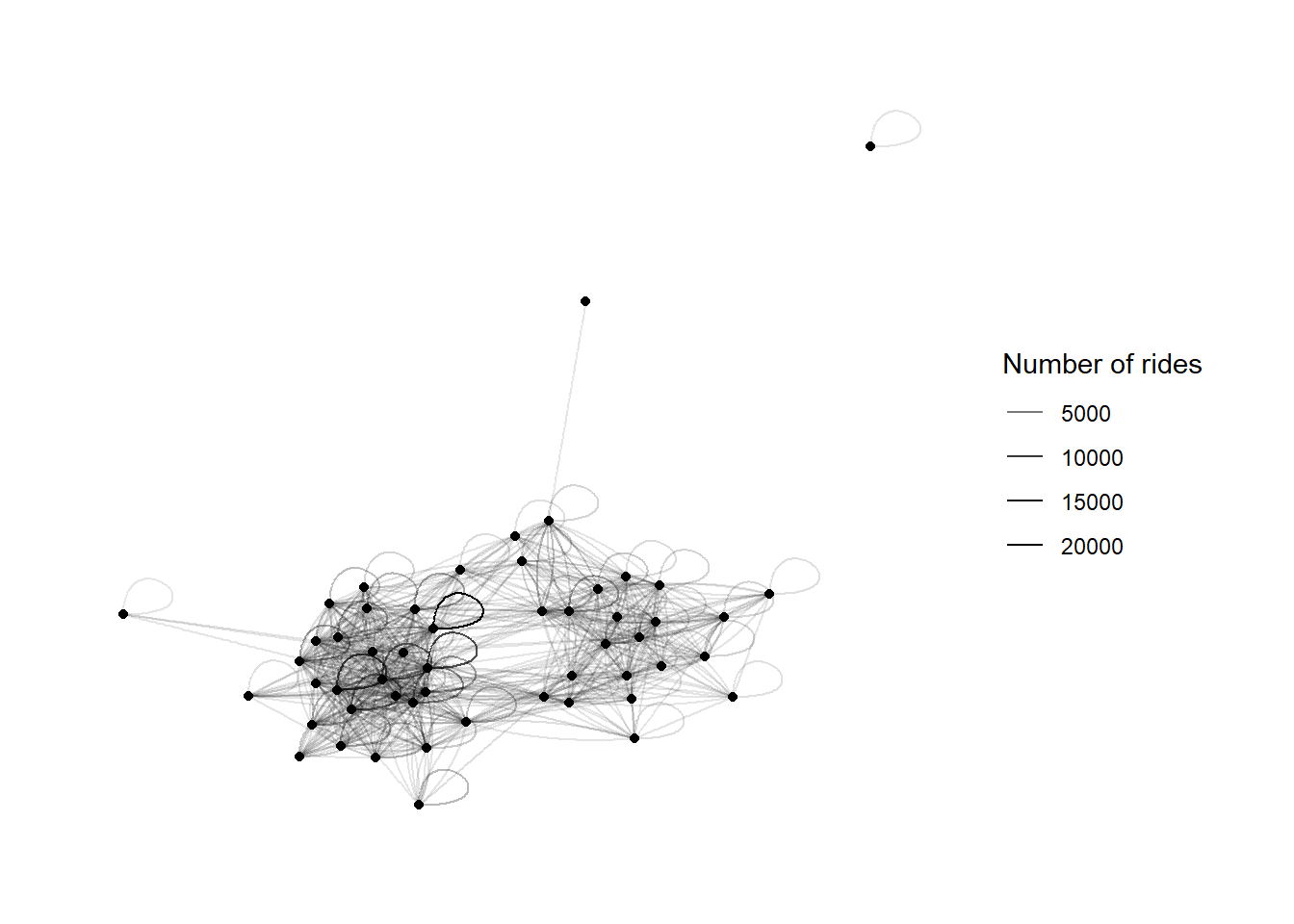There appear to be two clusters of stations. How does the network appear without trips that start and end at the same station? simple_network <- data_wide %>% count(from_station_name, to_station_name, sort = TRUE) %>% filter(n >= 150, from_station_name != to_station_name) simple_network <- simple_network %>% graph_from_data_frame(directed = TRUE) ggraph(simple_network) + geom_edge_fan(aes(edge_alpha = n)) + geom_edge_loop(aes(edge_alpha = n)) + geom_node_point() + scale_edge_alpha_continuous("Number of rides", range = c(.1, 1))The two clusters stand out more in this view. We can also do a more traditional heatmap of the departure and arrival stations: simple_network <- data_wide %>% count(from_station_name, to_station_name, sort = TRUE) %>% top_n(400) %>% ungroup() %>% complete(from_station_name, to_station_name) %>% replace_na(list(n = 0)) %>% mutate(from_station_name_abbr = str_c(str_sub(from_station_name, 1, 18), "..."), #abbreviate the station names so they fit on the axes to_station_name_abbr = str_c(str_sub(to_station_name, 1, 18), "...")) #check that we aren't losing any station names length(unique(simple_network$to_station_name)) == length(unique(simple_network\$to_station_name_abbr))
##  TRUE
#create vector for ordering the x axis
x_axis <- simple_network %>%
group_by(from_station_name_abbr) %>%
summarize(n = sum(n)) %>%
arrange(desc(n)) %>%
select(from_station_name_abbr) %>%
unlist()

#create vector for ordering the yaxis
y_axis <- simple_network %>%
group_by(to_station_name_abbr) %>%
summarize(n = sum(n)) %>%
arrange(desc(n)) %>%
select(to_station_name_abbr) %>%
unlist()

simple_network %>%
mutate(from_station_name_abbr = factor(from_station_name_abbr, levels = x_axis),
to_station_name_abbr = factor(to_station_name_abbr, levels = y_axis)) %>%
ggplot(aes(from_station_name_abbr, to_station_name_abbr, fill = n)) +
geom_tile() +
theme_bw() +
coord_equal() +
scale_fill_viridis() +
guides(fill = guide_colorbar(title = "Number of Rides")) +
theme(axis.text = element_text(size = 6),
axis.text.x = element_text(angle = 90, vjust = 0))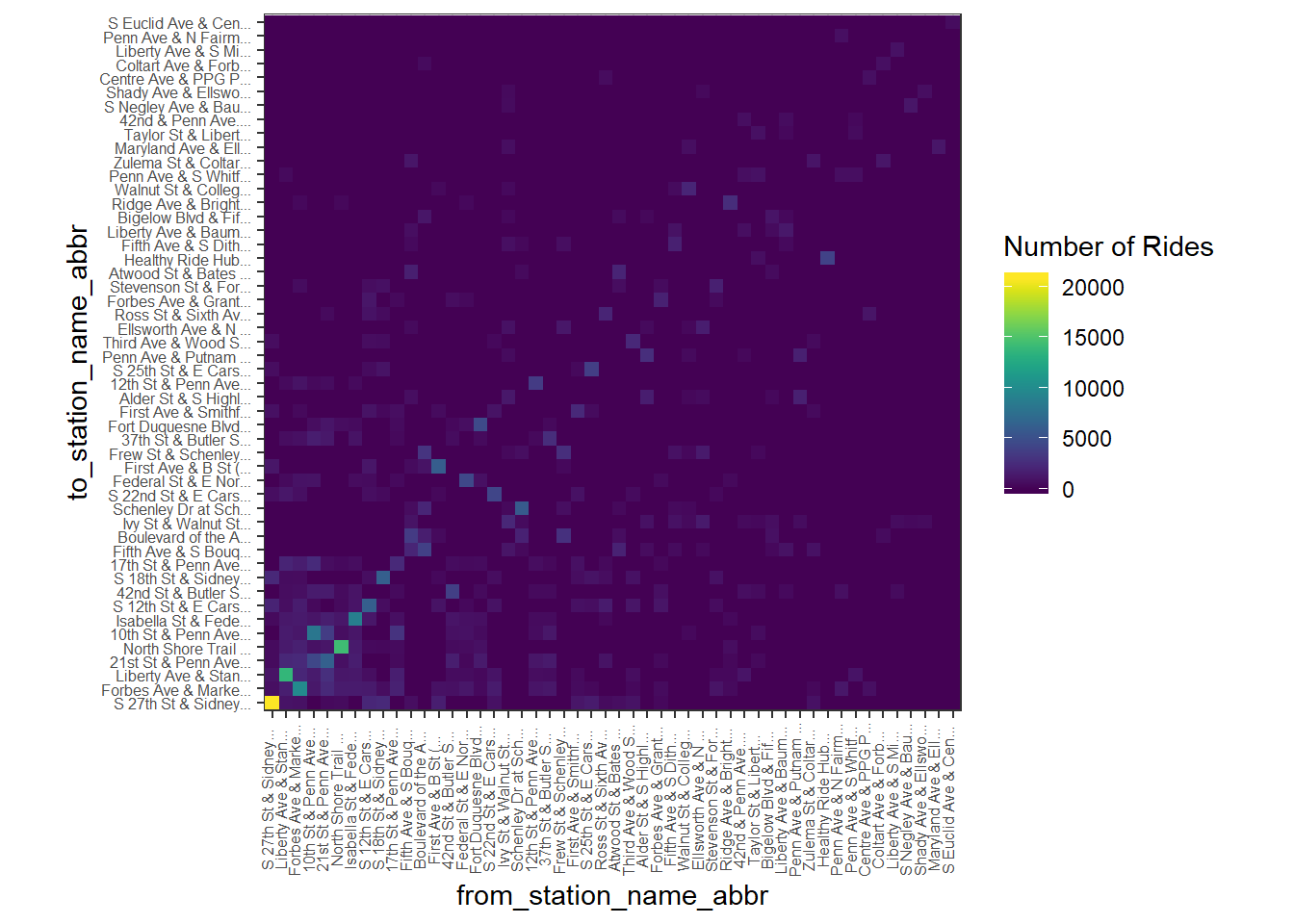It appears that many trips start and end at the same station.

We can investigate this phenomenon:

data_wide %>%
select(from_station_name, to_station_name) %>%
mutate(is_same = ifelse(from_station_name == to_station_name, "same_location", "different_location")) %>%
group_by(is_same) %>%
count() %>%
gather(location_type, n) %>%
select(location_type, n) %>%
mutate(n = round(n / sum(n), digits = 2)) %>%
kable("html") %>%
kable_styling()
location_type n
different_location 0.73
same_location 0.27

27% of all trips end at the same station they began at.

Does this pattern differ on weekdays vs. weekends?

df_test <- data_wide %>%
select(from_station_name, to_station_name, is_weekday) %>%
mutate(is_same = ifelse(from_station_name == to_station_name, "same_location", "different_location")) %>%
group_by(is_same, is_weekday) %>%
count() %>%
gather(location_type, n, -is_weekday) %>%
mutate(from_location = "from_location") %>%
select(is_weekday, from_location, location_type, n) %>%
mutate(n = round(n / sum(n), digits = 2)) %>%
arrange(is_weekday)

df_test %>%
ggplot(aes(is_weekday, n, fill = location_type)) +
geom_col(position = "dodge", color = "black") +
scale_y_continuous(labels = scales::percent) +
labs(y = "% of rides",
title = "") +
guides(fill = guide_legend(title = "Location Type")) +
theme_bw()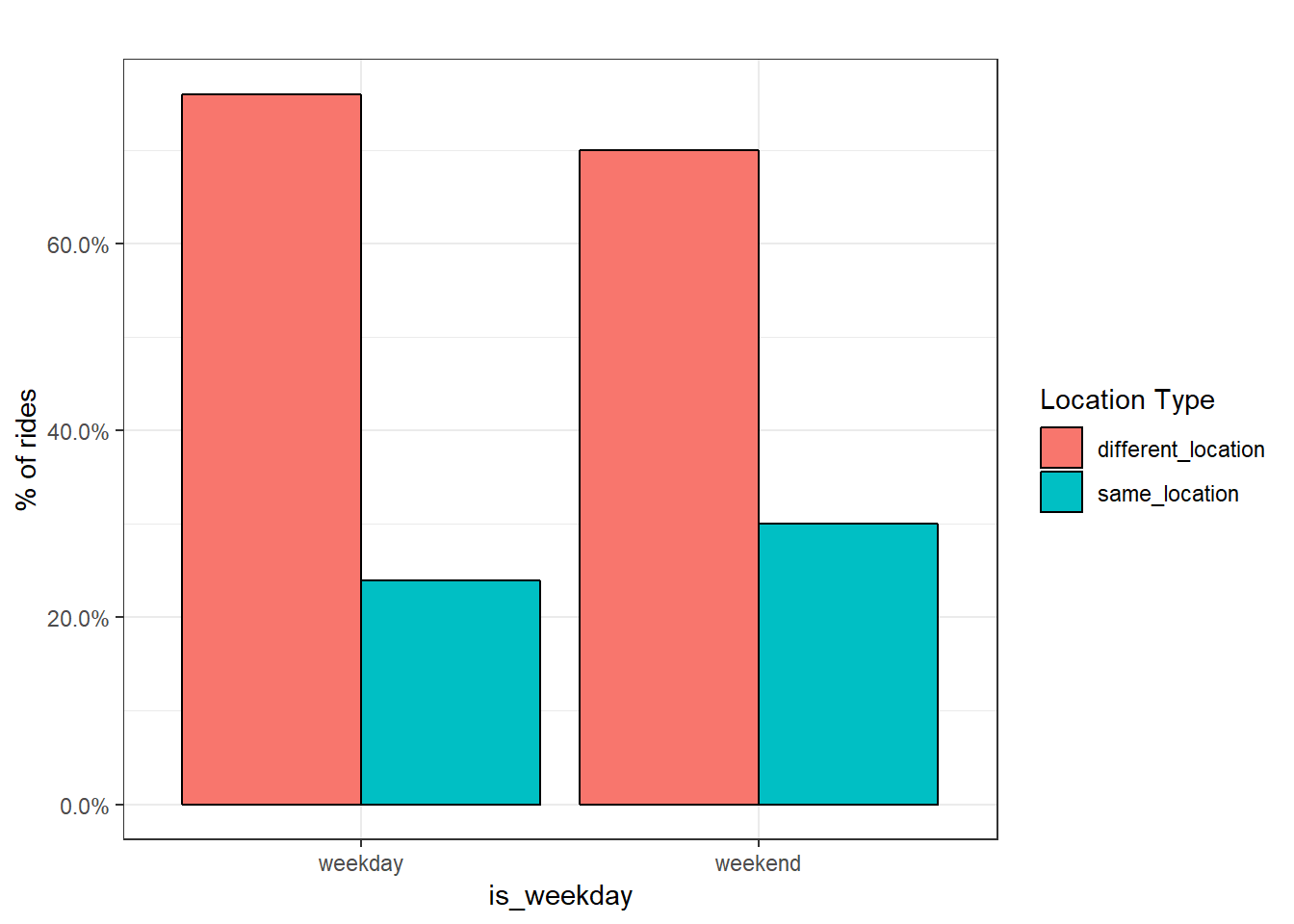A greater percentage of trips start and end at the same station on the weekends than on weekdays. This could be caused by people commuting to bike stations via car, going for a ride on a bike trail, and then returning to the same station.

Does the network look different on weekdays vs. weekends?

simple_network <- data_wide %>%
count(from_station_name, to_station_name, is_weekday, sort = TRUE) %>%
filter(n >= 150)

simple_network <- simple_network %>%
graph_from_data_frame(directed = TRUE)

ggraph(simple_network) +
geom_edge_fan(aes(edge_alpha = n,
color = is_weekday)) +
geom_edge_loop(aes(edge_alpha = n,
color = is_weekday)) +
geom_node_point() +
scale_edge_alpha_continuous("Number of rides", range = c(.3, 1)) +
scale_edge_color_discrete("Type of day") +
facet_edges(~is_weekday) +
th_foreground(foreground = 'grey80', border = TRUE)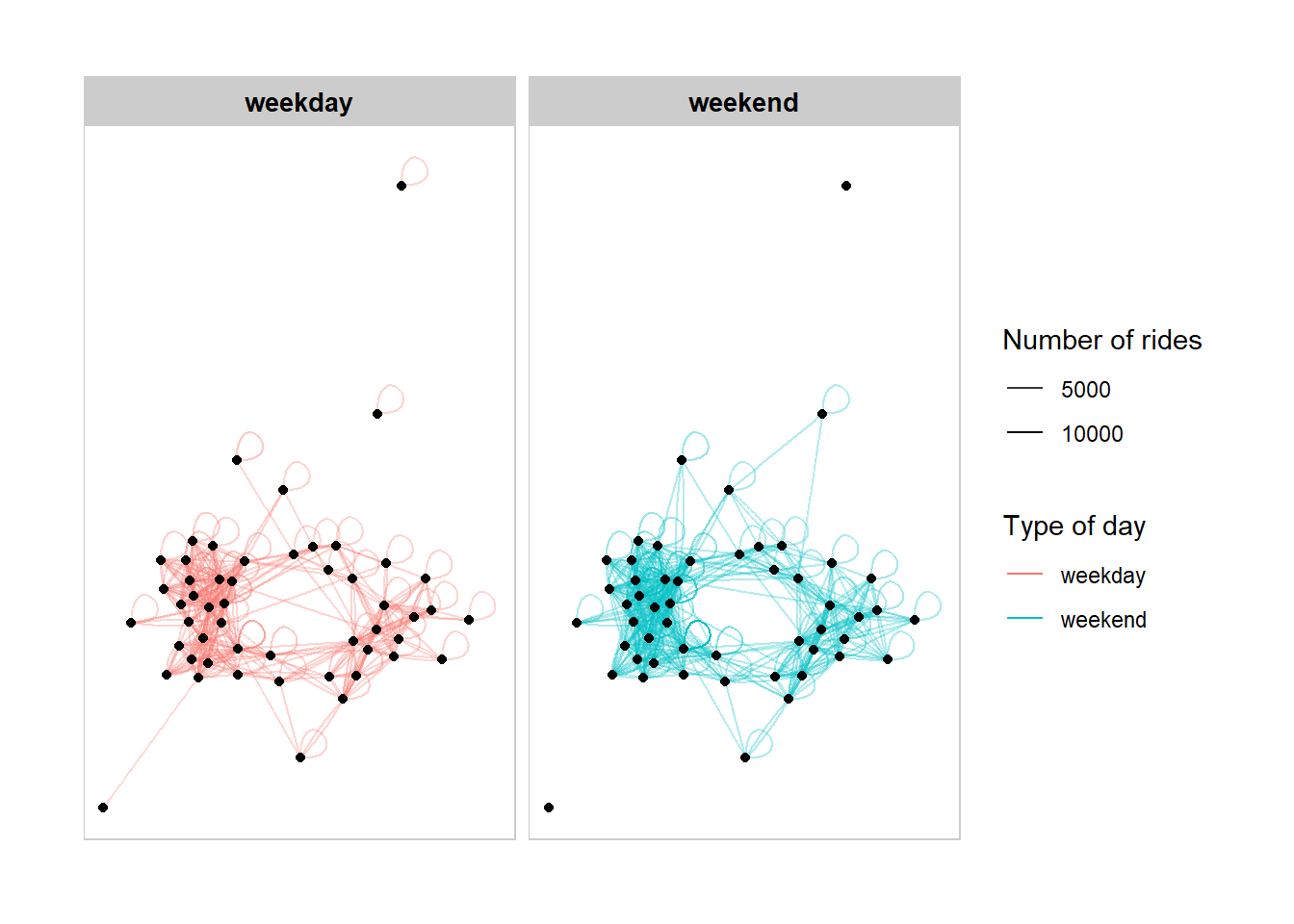The secondary cluster (on the bottom right) appears to be less connected on weekends